Accessing OP Malhotra Class 9 Maths Solutions Chapter 8 Triangles Ex 8(C) can be a valuable tool for students seeking extra practice.

## S Chand Class 9 ICSE Maths Solutions Chapter 8 Triangles Ex 8(C)

Question 1.
In a ∆PQR, side PQ is produced to S so that QS = RQ. If ∠PQR = 60° and ∠RPQ = 70°, prove that
(i) PS > RS
(ii) PS > PR
Solution:
Given : In the figure, ∆PQR, whose side PQ is produced to S such that QS = RQ
∠PQR = 60° and ∠RPQ = 70°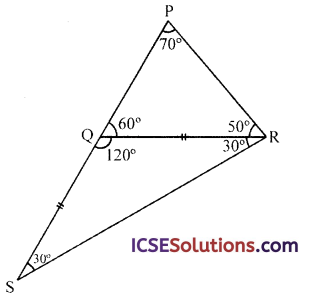To prove :
(i) PS > RS
(ii) PS > PR
Proof: In ∆PQR,
∵ ∠PQR = 60° and ∠RPQ = 70°
∴ ∠QRP = 180° – (60° + 70°)
= 180° – 130° = 50°
and Ext. ∠RQS =180° – 60° = 120°
∵ In ∆RSQ,
QS = QR (given)
∴ ∠QSR = ∠QRS
But ∠QSR + ∠QRS + ∠RQS = 180°
∴ ∠QSR + ∠QRS + 120°= 180°
⇒ ∠QSR + ∠QRS =180°- 120° = 60°
∴ ∠QSR = ∠QRS = $$\frac { 60° }{ 2 }$$ = 30°

(i) In ∆PSR,
∠PRS = ∠PRQ + ∠QRS = 50° + 30° = 80°
∵ ∠PRS > ∠RPS
∴ PS > RS
(∵ side opposite to greater angle is greater)

(ii) Similarly
∵ ∠PRS > ∠PSR (∵ ∠PSR = 30°)
∴ PS > PR
Hence proved.Question 2.
In a ∆PQR, ∠Q = 35°, ∠R = 61°, the bisector of ∠QPR cuts QR at X. Arrange in descending order PX, QX, RX.
Solution:
Given : In ∆PQR, PX is the bisector of ∠P meeting QR at X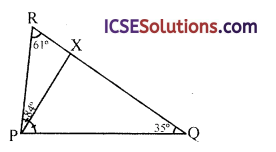∠Q = 35°, ∠R = 61°
∴ ∠P= 180° – (35° + 61°)
= 180° – 96° = 84°
∵ PX is the bisector of ∠QPR
∴ ∠RPX = ∠QPX = $$\frac { 84° }{ 2 }$$ = 42°
Now ∠PXQ = ∠RPX + ∠PRQ
= 42° + 61° = 103°
and ∠PXR = 180° – ∠PXQ = 180° – 103° = 77°
Now we have to arrange PX, QX and RS in descending order
In ∆PXQ, > PQS (42° > 35°)
In ∆PXQ, QPX > PQS
∴ XQ > PX … (i)
and in ∆PRX,
∠PRX > ∠RPX (∵ 610 > 42°)
∴ XP > XR … (ii)
From (i) and (ii),
XQ > PX > XR
⇒ QX > PX > RX
Which are in descending order

Question 3.
In the figure, AB < BC. If the base angles of ∆ABC are 70° and 50°, state which of the two is 70°. AM bisects the exterior angle BAX and AP is parallel to CB, find ∠MAP.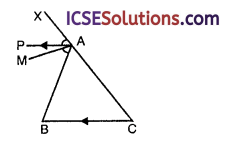Solution:
Given : In ∆ABC,
AB < BC
and base angles are 70° and 50°
AM is the bisector of ∠BAX and AP || BC
Now we have to find ∠MAP
∵ AB < BC
∴ ∠C < ∠B
∴ ∠C will be = 50° and ∠B = 70°
(angles opposite to greater side is greater)
In ∆ABC
Ext. BAX = ∠B + ∠C
= 70°+ 50° = 120°
∵ AM is the bisector of ∠BAX (given)
∠MAX = ∠MAB = $$\frac { 120° }{ 2 }$$ = 60°
∴ ∠PAB = ∠ABC = 70°
Now ∠MAP = ∠PAB – ∠MAB
= 70° – 60° = 10°

Question 4.
In the figure, ABCD is a straight line which is greater?
(i) RB or RC
(ii) PB or PR?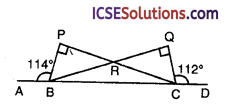Solution:
In the figure, ∆BCD is a straight line
∠BPR = ∠CQR = 90°, ∠ABP =114° and ∠DCQ = 112°
To find : Which is greater
(i) RB or RC
(ii) PB or PR?
(i) In ∆BPR, ∠P = 90° and in ∠CQR = 90°
∠PRB = ∠QRC (vertically opposite angles)
∠PBR = ∠QCR
But ∠PBA + ∠PBC = 180° (linear pair)
⇒ 114° +∠PBC = 180°
⇒ ∠PBC = 180°- 114° = 66°
Similarly ∠QCB = 180° – 112° = 68°
∴ ∠QCB > ∠PBC ⇒ ∠QCR + ∠RCB> ∠PBR + ∠RBC
But ∠PBR = ∠QCR (proved)
∴ ∠RCB > ∠RBC
∴ RB > RC

(ii) ∵ ∠PBR > ∠PRB
∴ PR > PB

Question 5.
In the figure, arrange the angles in descending order of magnitude.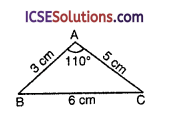Solution:
In AABC, AB = 3 cm, AC = 5 cm and BC = 6 cm
∴ ∠A > ∠B and ∠A > ∠C
But AC > AB
∴ ∠B > ∠C
∴ ∠A > ∠B > ∠C or
∴ ∠A, ∠B and ∠C are in descending order

Question 6.
In the figure, which is longer
(i) LM or MN
(ii) PQ or PR
(iii) AB || DC; AB or BD
(iv) PB or PC, given PB and PC bisect ∠ABC and ∠ACB respectively.
(v) QM or QR if LM > LR and ∠LMQ = ∠LRQ.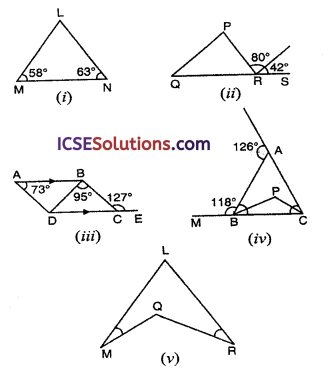Solution:
(i) In ∆LMN,
∠M = 58°, ∠N = 63°
∴ ∠L = 180° – (58° + 63°)
= 180° – 121° = 59°
∵ ∠N > ∠L
∴ LM > MN
or LM is longer

(ii) In ∆PQR,
∵ RT || QP
∴ ∠PQR = ∠TQS (corresponding angles)
= 42°
and ∠QPR = ∠PRT (alternate angles)
= 80°
∴ ∠PRQ = 180° – (80° + 42°) = 180° – 122°
= 58°
∵ ∠PRQ > ∠PQR
∴ PQ > PR
∴ PQ is longer

(iii) ABCD is a quadrilateral and DC is a produced to E
∠BCE = 127°
∠A = 73° and ∠CBD = 95°
∵ ∠BCD + ∠BCE = 180° (linear pair)
∴ ∠BCD = 180°- 127° = 53°
∴ ∠BDC = 180° – (95° + 53°) = 180° – 148°
= 32°
⇒ ∠ADB + 32°= 127°(corresponding angles)
⇒ ∠ADB = 127° – 32° = 95°
∴ BA > BD
BA is longer(iv) In the ∆ABC,
PB and PC are the bisectors of
∠ABC and ∠ACB respectively
Ext. ∠LAB = 126° and ∠MBA = 118°
Now ∠ABC + ∠ABM = 180° (linear pair)
⇒ 118° + ∠ABC = 180° ⇒ ∠ABC = 180° – 118° = 62°
∴ ∠PBC = $$\frac { 1 }{ 2 }$$ ∠ABC = $$\frac { 1 }{ 2 }$$ x 62° = 31°
and ∠BAL = ∠ABC + ∠ACB = 126°
⇒ 126° = 62° +∠ACB
⇒ ∠ACB = 126° – 62° = 64°
∴ ∠PCB = $$\frac { 1 }{ 2 }$$ ∠ACB = $$\frac { 1 }{ 2 }$$ x 64° = 32°
∴ In ∆PBC
∠PCB > ∠PBC
∴ PB > PC
or PB is longer

(v) In the figure,
LM > LR and
∠LMQ = ∠LRQ
∵ LM > LR
∴ ∠LRM > ∠LMR
(angle opposite to greater side is greater)
But ∠M = ∠R (given)
∴ ∠QRM > ∠QMR
∴ QM > QR
Hence QM is longerQuestion 7.
(a) If three sides of a triangle are 3, 4 and 5, then the greatest angle is opposite to the side 5 units.
(b) If two sides of a triangle are unequal, the greater side has the greater angle’ opposite to it.
(c) The sides of a certain triangle are 36,46 and 84 cm.
Solution:
(a) Three sides of a ∆ are 3, 4, 5 units in which side of 5 units is greater
∴ angle opposite to it greater Yes
(b) True : Greater side of a triangle has greater angle opposite to it.
(c) Sides of a triangle are 36, 46 and 84 cm It is not possible as
36 + 46 = 82
and 82 > 84
as sum of any two sides of a triangle is greater than the third side.

Question 8.
The side BC of a triangle ABC is produced to D, so that CD = AC. If the angle BAD = 109° and the angle ACD = 72°, prove that BC is greater than AC.
Solution:
Given : In ∆ABC, BC is produced to D such that CD = AC
∠BAD = 109° and ∠ACD = 72°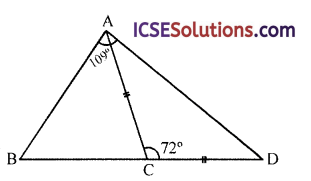To prove : BC > AC
Proof: ∵ AC = CD
But ∠CAD + ∠CDA + ∠ACD = 180°
(angles of a triangle)
⇒ ∠CAD + ∠CDA + 72° = 180°
⇒ ∠CAD + ∠CDA =180° – 72° = 108°
∴ ∠CAD = ∠CDA =$$\frac { 108° }{ 2 }$$ = 54°
∴ ∠BAC = ∠BAD – ∠CAD = 109° – 54° = 55°
and ∠B = 180° – (∠A + ∠D) = 180° – (109° + 54°)
∠B = 180° – 163° = 17°
∵ ∠BAO > ∠B
∴ BC > AC
Hence proved.

Question 9.
Can you draw triangle with sides (i) 5 cm, 7 cm, 8 cm (ii) 2.5 cm, 1 cm, 3.5 cm (iii) 2 cm, 3 cm, 5.6 cm (iv) 3.5 cm, 3.5 cm, 4.1 cm Give reasons in each case.
Solution:
We know that in a triangle,
Sum of any two sides is greater than the third side
(i) In triangle sides are 5 cm, 7 cm, 8 cm
∵ 5 + 7= 12
and 12 > 8 (third side)
∴ This triangle can be drawn

(ii) Here 2.5 cm + 1 cm = 3.5 cm
and 3.5 cm = 3.5 cm (third side)
∴ It can not be drawn

(iii) 2 + 3 = 5 cm
and 5 cm < 5.6 cm
∴ It cannot be drawn

(iv) 3.5 + 3.5 = 8.0 cm
and 7.0 > 4.1 cm (third side)
∴ This triangle can be drawn

Question 10.
In the figure, XY is a diameter. Prove that XY > XZ.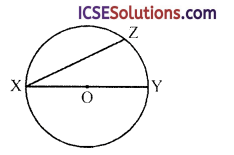Solution:
Given : In the figure, XOY is the diameter of the circle XZ is any chord
To prove : XY > XZ
Construction : Join ZXProof:
∵ XOY is the diameter of the circle
∴ ∠XZY = 90° (angle in a semicircle)
∵ ∠ZXY + ∠ZYX = 90°
or ∠XZY > ∠XYZ and also > ∠ZXY
∴ XY > XZ
Hence proved.

Question 11.
In the figure, prove that AH < HC and DC > DH.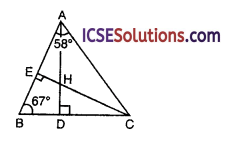Solution:
Given: In ∆ABC,
AD ⊥ BC, CE ⊥ AB
∠BAC = 58°, ∠ABC = 67°
AD and CE intersect each other at H To prove :
(i) AH < HC
(ii) DC > DH
Proof:
In ∆AEC,
∠AEC = 90°, ∠CAE = 58°
∴ ∠ACE = 180° – (90° + 58°) = 180° – 148° = 32°
∠ADB = 90°, ∠ABD = 69°
∴ ∠BAD =180°- (90° + 67°) = 180° – 157° = 23°
∠CAD = 58° – 23° = 35°
Now in ∆AHC,
∠ACE or ∠ACH < ∠CAD or ∠CAH
∴ AH < HC

(ii) In ∆HDC
∠D = 90°
∴ ∠DHC + ∠DCH = 90°
In ∆BCE, ∠E = 90°
∴ ∠BCE = 90° – 67° = 23°
∴ In ∆DHC,
∠DHC = 90° – ∠BCE
= 90° – ∠DCH = 90° – 23° = 67°
∵ ∠DHC > ∠HCD
∴ DC > DH
Hence proved.Question 12.
PQRS is a convex quadrilateral. Prove that PQ + QR + RS > PS.
Solution:
Given : PQRS is a convex quadrilateral
To prove : PQ + QR + RS > PS
Construction : Join PR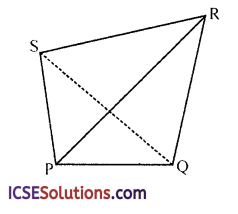Proof: We know that sum of any two sides of a triangle is greater than its third side
∴ In ∆PQR
PQ + QR > PR … (i)
and in APRS
PR + RS > PS … (ii)
From (i) and (ii)
PQ + QR + RS > PS
Hence proved.

Question 13.
In the figure, M is any point inside the triangle PQR. PM is produced to meet QR in N. Prove that
(i) ∠QMN > ∠QPN
(ii) ∠QMR > ∠QPR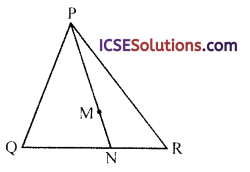Solution:
Given : In ∆PQR, M is any point inside the triangle. PM is.joined and produced to meet QR in N
To prove :
(i) ∠QMN > ∠QPN
(ii) ∠QMR > ∠QPR
Construction : Join MQ and MRProof:
(i) In ∆PQM,
Ext. ∠QMN = ∠MQP + ∠QPM
∴ ∠QMN > ∠QPM or ∠QPN … (i)

(ii) Similarly in ∆PMR,
Ext. ∠RMN > ∠RPM or ∠RPN … (ii)
∠QMN + ∠RMN > ∠QPN + ∠RPN
⇒ ∠QMR > ∠QPR
Hence proved.

Question 14.
In the figure, if ∠1 = ∠2, prove that BA > BD.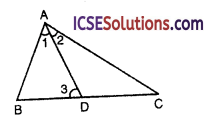Solution:
Given : In the figure, in ∆ABC,
AD is the bisector of ∠A
i.e., ∠1 = ∠2
To prove : BA > BD
Ext. ∠3 > its interior opposite angle∠2
But ∠2 = ∠1 (AD is bisector of ∠A)
∴ ∠3 > ∠1
Now in ∆ABD
∵ ∠3 > ∠L
∴ AB > BD
(side opposite to greater angle is greater)
or BA > BD
Hence proved.

Question 15.
In figure, ABC is a triangle in which AC > AB and the bisectors of angles B and C intersect each other at O. Prove that OC > OB.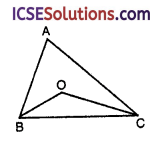Solution:
Given : In ∆ABC, AC > AB
Bisectors of ∠B and ∠C meet each other at O
To prove : OC > OB
Proof: In ∆ABC,
∵ AC > AB (given)
∴ ∠B > ∠C
(angle opposite to greater side is greater)
⇒ $$\frac { 1 }{ 2 }$$ < B > $$\frac { 1 }{ 2 }$$ < C ⇒ ∠OBC > ∠OCB
(∵ BO and CO are bisectors of ∠B and ∠C)
∴ OC > OB
(side opposite to greater angle is greater)
Hence proved.

Question 16.
In the figure, If AB = AC, then prove that CD > BD.Solution:
Given : In figure AB = AC
To prove : CD > BD
Proof: In ∆ABC,
AB = AC (given)
∴ ∠ABC = ∠ACB
Now ∠CBD = ∠ABC + ∠DBA
In ∆DBC,
∴ ∠CBD > ∠ABC
⇒ ∠CBD > ∠ACB (∵ ∠ABC = ∠ACB)
∴ CD > BD
(side opposite to greater angle is greater)
Hence proved.Question 17.
Diagonals PR and QS of a quadrilateral PQRS intersect each other at O.
Prove that:
(i) PQ + QR + RS + SP > PR + QS
(ii) PQ + QR + RS + SP < 2 (PR + QS)Solution:
Given : In quadrilateral PQRS, diagonals PR and QS intersect each other at O
To prove:
(i) PQ + QR + RS + SP > PR + QS
(ii) PQ + QR + RS + SP < 2 (PR + QS)
Proof:
In ∆PQR,
PQ + QR > PR … (i)
(sum of any two sides of a triangle is greater than the third side)
Similarly in ∆PRS
RS + SP > PR … (ii)
PQ + QR + RS + SP > PR + PR
⇒ PQ + QR + RS + SP > 2PR … (iii)
Similarly we can proved that
PQ + QR + RS + SP > 2QS (iv)
2 (PQ + QR + RS + SP) > 2 (PR + QS)
⇒ PQ + QR + RS + SP > PR + QS

(ii) In ∆OPQ,
OP + OQ > PQ … (a)
Similarly in ∆QOR
OQ + OR > QR … (b)
In ∆ROS,
OR + OS > RS …(c)
and in ∆SOP,
OS + OP > SP … (d)
OP + OQ + OQ + OR + OR + OS + OS + OP > PQ + QR + RS + SP
⇒ 2 (OP + OQ + OR + OS) > PQ + QR + RS + SP
⇒ 2 (PR + QS) > PQ + QR + RS + SP
⇒ PQ + QR + RS + SP < 2 (PR + QS)
Hence proved.

Question 18.
Prove that any two sides of a triangle are together greater than the third side.
Solution:
Given: ∆ABC
To prove : AB + AC > BC
AC + BC > AB
BC + AB > AC
Construction: Produce BA to D such that
Join CDProof: In ∆ACD,
(opposite angle to equal sides)
∴ BD > BC ⇒ BA + AD > BC
⇒ AB + AC > BC (∵ AD = AC)
Similarly we can prove that
AC + BC > AB and BC + AB > AC

Question 19.
Prove that in a triangle, the difference of any two sides is less than the third side.Solution:
Given : ∆ABC, AC > AB
To prove : AC – AB < BC
Construction : From AC, cut off AD = AB
Join BD
Proof: In ∆ABC, CD = AC – AD = AC – AB
Now AB + BC > AC ⇒ AB + BC > AD + DC
BC > DC (∵ AB = AD)
⇒ BC > AC – AB
Hence proved.